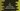# C program to find the sum of first n natural numbers## C program to find the sum of first n natural numbers:

In this post, we will learn how to find the sum of the first n natural numbers. The program will take the value of n as an input from the user and it will print the sum of the first n numbers.

We can solve this problem in different ways. We have to run one loop. We can use any loop we want to use.

### Algorithm to use:

We will use the below algorithm to solve this program:

• Take the value of n as an input from the user.
• Initialize a sum variable as 0 to hold the final sum.
• Run one loop from 1 to n and on each iteration of the loop, add it to the sum variable.
• Once the loop will end, print the value of sum which is the final sum.

### Method 1: C program with a for loop:

Let’s write the program with a for loop.

``````#include <stdio.h>

int getSum(int n)
{
int sum = 0;
for (int i = 1; i <= n; i++)
{
sum += i;
}
return sum;
}

int main()
{
int n;

printf("Enter the value value of n: ");
scanf("%d", &n);

printf("Sum of 1 to %d: %d\n", n, getSum(n));
}``````
• This program asks the user to enter the value of n
• It calls the method getSum to get the sum from 1 to n. It takes the value of n as the parameter.
• The last line is printing the sum value.
``````Enter the value value of n: 5
Sum of 1 to 5: 15``````

### Method 2: By using a while loop:

We can also use a while loop to write the above program.

``````#include <stdio.h>

int getSum(int n)
{
int sum = 0, i = 1;
while (i <= n)
{
sum += i;
i++;
}
return sum;
}

int main()
{
int n;

printf("Enter the value value of n: ");
scanf("%d", &n);

printf("Sum of 1 to %d: %d\n", n, getSum(n));
}``````

Here, we replaced the for loop with a while loop. It works in a similar way. The difference is that it initialises the value of i before the loop starts and the value of i is incremented at the end of each iteration of the loop.

If you run this program, it will give a similar output.

### Method 3: By using a do-while loop:

We can also use a do-while loop to write the same program. The while loop checks the condition and runs the code of its body. But, do-while loop runs the body and then it checks the condition.

``````#include <stdio.h>

int getSum(int n)
{
int sum = 0, i = 1;
do
{
sum += i;
i++;
} while (i < n + 1);
return sum;
}

int main()
{
int n;

printf("Enter the value value of n: ");
scanf("%d", &n);

printf("Sum of 1 to %d: %d\n", n, getSum(n));
}``````

We will have to change the condition to i < n + 1 because the value of i is incremented by 1 at the end of each iteration and then the condition is checked.

### Method 4: With mathematical formula:

There is a mathematical formula to calculate the sum of the first n numbers. This is:

``n * (n + 1)/2``

We don’t have to run a loop and it is faster than any other method mentioned above.

Let me write down the C program:

``````#include <stdio.h>

int getSum(int n)
{
return n * (n + 1) / 2;
}

int main()
{
int n;

printf("Enter the value value of n: ");
scanf("%d", &n);

printf("Sum of 1 to %d: %d\n", n, getSum(n));
}``````

If you run it, it will give similar outputs.